Courses

# Solutions of Refraction of Light (Page No- 220) - Physics By Lakhmir Singh, Class 10 Class 10 Notes | EduRev

## Class 10 : Solutions of Refraction of Light (Page No- 220) - Physics By Lakhmir Singh, Class 10 Class 10 Notes | EduRev

The document Solutions of Refraction of Light (Page No- 220) - Physics By Lakhmir Singh, Class 10 Class 10 Notes | EduRev is a part of the Class 10 Course Class 10 Physics Solutions By Lakhmir Singh & Manjit Kaur.
All you need of Class 10 at this link: Class 10

Lakhmir Singh Physics Class 10 Solutions Page No:220

Question 13: A ray of light passes from air into a block of glass. Does it bend towards the normal or away from it ?

Solution : A ray of light travelling from air to glass block, will bend towards the normal.

Question 14: As light rays pass from water into glass, are they refracted towards the normal or away from the normal ?

Solution : A ray of light travelling from water into glass will bend towards the normal.

Question 15: In which material do you think light rays travel faster-glass or air ?

Solution : Light rays travel faster in air.

Question 16: Which phenomenon of light makes the water to appear shallower than it really is ?

Solution : Refraction of light.

Question 17: State whether the following statement is true or false :

Refraction occurs because light slows down in denser materials.

Solution : True.

Question 18: Why does a ray of light bend when it travels from one medium to another ?

Solution : A ray of light bends when it travels from one medium to another due to the change in the speed of light.

Question 19: Fill in the following blanks with suitable words :
(a) Light travelling along a normal is…………
(b) Light bends when it passes from water into air. We say that it is……………

Solution : (a) not.
(b) refracted.

Question 20: What is meant by ‘refraction of light’ ? Draw a labelled ray diagram to show the refraction of light.

Solution : The change in direction of light when it passes from one medium to another obliquely, is called refraction of light.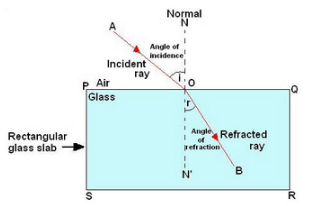Question 21: A ray of light travelling in air is incident on a rectangular glass block and emerges out into the air from the opposite face. Draw a labelled ray diagram to show the complete path of this ray of light. Mark the two points where the refraction of light takes place. What can you say about the final direction of ray of light ?

Solution :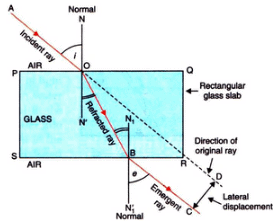The final direction of the ray of light is same as the incident direction.

Question 22: Draw a labelled ray diagram to show how a ray of light is refracted when it passes :
(a) from air into an optically denser medium.
(b) from an optically denser medium into air.

Solution : (a) Ray of light travelling from air into an optically denser medium.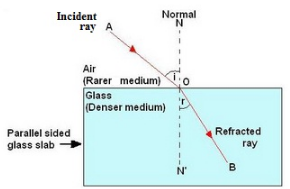(b) Ray of light travelling from an optically denser medium into air.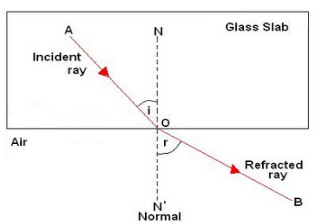Question 23: The diagram given alongside shows a ray of light entering a rectangular block of glass.
(a) Copy the diagram and draw the normal at the point of entry.
(b) Draw the approximate path of the ray of light through the glass block and out of the other side.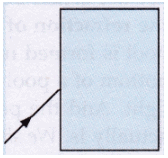Solution :

(a)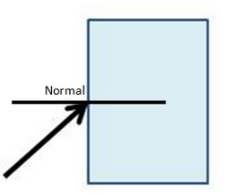(b)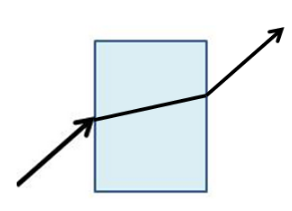Question 24: What is meant by the ‘angle of incidence’ and the ‘angle of refraction’ for a ray of light ? Draw a labelled ray diagram to show the angle of incidence and the angle of refraction for a refracted ray of light.

Solution : The angle between the incident ray and normal at the point of incidence is called angle of incidence. The angle between the refracted ray and normal at the point of refraction is called angle of refraction.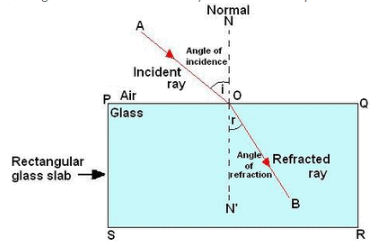Question 25: Light travels more quickly through water than through glass.
(a) Which is optically denser : water or glass ?
(b) If a ray of light passes from glass into water, which way will it bend : towards the normal or away from the normal ?

Solution : (a) Glass is optically denser than the water.
(b) The ray will bend away from the normal.

Question 26: Draw a labelled ray diagram to show how a ray of light passes through a parallel sided glass block :
(a) if it hits the glass block at 90° (that is, perpendicular to the glass block)
(b) if it hits the glass block at an angle other than 90° (that is, obliquely to the glass block).

Solution : (a) If ray of light hits the block at 90°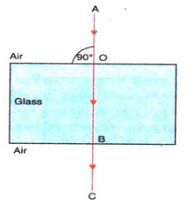(a) If ray of light hits the block other than the 90°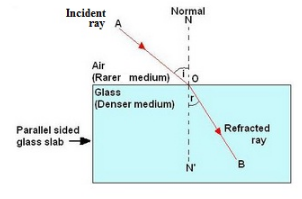Question 27: When a light ray passes from air into glass, what happens to its speed ? Draw a diagram to show which way the ray of light bends.

Solution :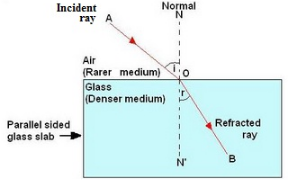The ray of light bends towards the normal.

Question 28: (a) Explain why, a stick half immersed in water appears to be bent at the surface. Draw a labelled diagram
(b) A coin in a glass tumbler appears to rise as the glass tumbler is slowly filled with water. Name the phenomenon responsible for this effect.

Solution : (a) The apparent bending of the stick is due to the refraction of light when it passes from water into air.
A ray of light OC coming from O passes from water into air and gets refracted away from normal (along CX). Another ray OD gets refracted along DY. The two refracted rays CX and DY, when produced backwards, appear to meet at point I. Thus, I is the virtual image of the end O of the stick. So, the stick appears to be bent as shown below.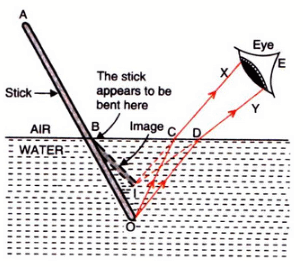(b) This phenomenon is due to the refraction of light as it comes out from water into air?

Question 29: (a) With the help of a labelled diagram, explain why a tank full of water appears less deep than it actually is.
(b) Name the phenomenon due to which a pencil partly immersed in water and held obliquely appears to be bent at the water surface.

Solution : (a) If we look into a tank of water, it appears to be less deep than it really is. This is due to the refraction of light which takes place when light rays pass from the tank of water into air. When we look into the tank, we do not see the actual bottom of the tank, we see a virtual image of the bottom of the pool which is formed by the refraction of light coming from the water into the air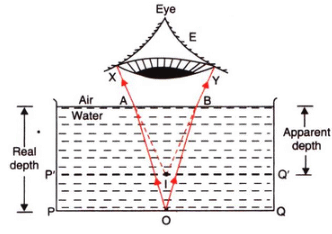(b) Refraction of light.

Question 30: (a) With the help of a diagram, show how when light falls obliquely on the side of a rectangular glass slab,
the emergent ray is parallel to the incident ray.
(b) Show the lateral displacement of the ray on the diagram.
(c) State two factors on which the lateral displacement of the emergent ray depends.

Solution :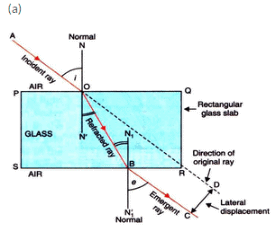(b) The lateral displacement is shown in the above diagram.
(c) Factors on which the lateral displacement depends are:
(i) Angle of incidence
(ii) Thickness of glass slab
(iii) Refractive index of glass slab

Question 31: Explain with the help of a labelled ray diagram, why a pencil partly immersed in water appears to be bent at the water surface. State whether the bending of pencil will increase or decrease if water is replaced by another liquid which is optically more dense than water.

Solution : A pencil placed in water appears to be bent because of refraction of light. The refraction causes an apparent shift in the position of the part of the pencil within the water.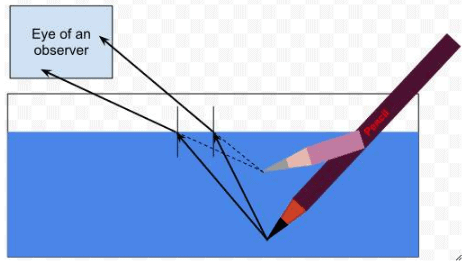If water is replaced by another liquid which is optically more dense than water, then the bending of the pencil will increase. This is because the optically denser medium will cause more refraction of light rays

Offer running on EduRev: Apply code STAYHOME200 to get INR 200 off on our premium plan EduRev Infinity!

94 docs

,

,

,

,

,

,

,

,

,

,

,

,

,

,

,

,

,

,

,

,

,

,

,

,

;